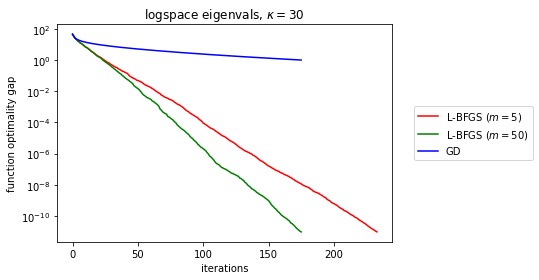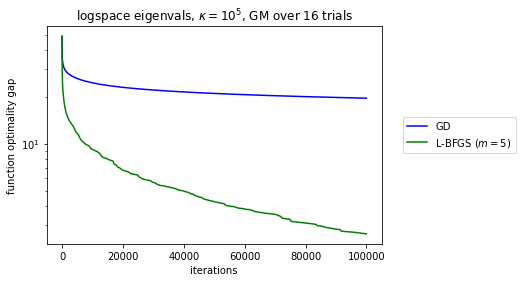# When does L-BFGS outperform GD?

In practice, L-BFGS is frequently held comparably to other inexact QN methods, and it provides a middle ground of sorts between Hestenes–Stiefel CG and BFGS as memory goes from zero to infinity (Numerical Optimization Ch. 7). Lots of empirical results show L-BFGS outperforms GD, at least in certain large classes of settings, like very strongly convex and smooth functions.

Liu and Nocedal 1989 prove that the L-BFGS descent direction angle with the gradient at the $$k$$-th iterate, $$\theta_k$$, satisfies the lower bound $$\cos^2\theta_k\ge \delta>0$$ eventually, which under moderate smoothness ensures GD-like convergence properties: since the descent agrees with GD enough, under line search satisfying Wolfe conditions, we get global eventual convergence and for smooth and strongly convex functions we achieve linear convergence.

However, the $$\cos^2\theta_k\ge \delta$$ result, the local and global convergence properties come from general theorems about any descent method with Wolfe conditions met. There's nothing special about L-BFGS. In fact, the inequalities used for the $$\cos^2\theta_k\ge \delta$$ result seem to get weaker as memory increases.

The linear local rate with worse constants is counter-intuitive and feels like a gap between theory and practice.

Are any theoretical results known where L-BFGS improves on GD's linear rate (or even its smoothness constants) in sufficiently regular settings, ideally in a way monotonically improving with memory use?

• In practice, L-BFGS does much better than GD in the earlier stages, long before the asymptotic behavior is apparent. Oct 26, 2020 at 18:10
• @BrianBorchers Very interesting! I've noticed the same for most QN methods, not just L-BFGS. Given counterexamples for BFGS convergence in the non-convex setting (Dai 2013) it's clear something special is going on in the "early stages" for this sufficiently regular but non-local regime.
– VF1
Oct 26, 2020 at 18:30
• At the very least, the BFGS matrix has the right physical units and ensures that the various quantities you add together in forming $x_{k+1} = x_k + \alpha_k p_k$ have the right units. This isn't true for the steepest/gradient descent method and so the result is quite sensitive to appropriate scaling of the variables in involved. Oct 26, 2020 at 23:27
• The performance of steepest descent with exact line search on quadratic problems is well studied- we know that convergence is asymptotically linear and know the rate constant. You might be able to show that the rate constant is strictly better for L-BFGS. Oct 28, 2020 at 4:18

(Not exactly an answer to my question, but some empirical experiments which might give guidance to what a worst-case analysis of the L-BFGS iteration of a quadratic function should result in). I tested directly translated algorithm pseudocode implementations of GD and L-BFGS. See the code and all my experiments here. Even in the nicest setting you can think of, a quadratic function with moderate condition number, L-BFGS exhibits no superlinearity. Generally speaking, it seems like L-BFGS behaves basically like a better-conditioned version of GD.

Here are some rapid-fire takeaways:

• L-BFGS convergence order looks linear regardless of memory
• L-BFGS, unsurprisingly, dominates GD in well-conditioned quadratic settings, owing to a much better linear rate.
• Interestingly, in my vanilla implementation, high-memory L-BFGS is unstable.
• Increases in memory seems to linearly improve the effective linear rate.
• The condition number exhibits the same $$(1-\kappa^{-1})$$-like behavior for the linear convergence rate for both L-BFGS and GD.Edit to answer Brian's question from the comments: With very high $$\kappa=10^5$$, scipy's inexact Wolfe line search fails to terminate for both GD and L-BFGS ($$m=5$$). Switching to Armijo I got noisy descents, so I resampled several different least squares problems with the same log-spaced singular values and geometrically averaged the resulting descent curves. The same pattern holds (eventually linear, L-BFGS dominates), but with much worse rates for everyone.• How does the comparison workout when $\kappa$ is much larger, say $10^{5}$? Nov 1, 2020 at 17:29
• @BrianBorchers see edit in post
– VF1
Nov 1, 2020 at 19:00